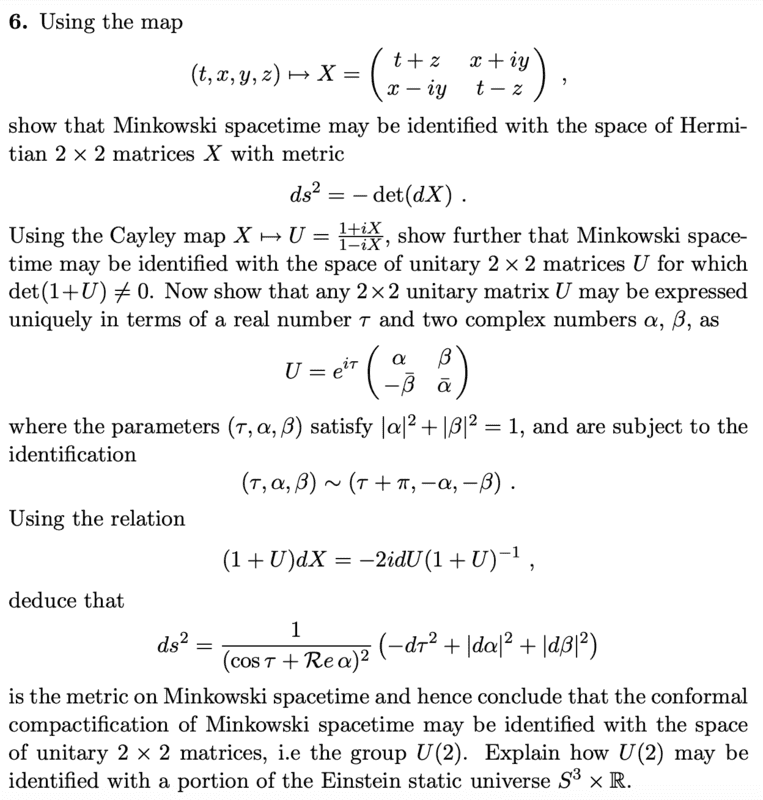# Minkowski spacetime as U(2)

etotheipi
Homework Statement:
It's question 6 of Example Sheet 1, very near the bottom of this document:
https://arxiv.org/pdf/gr-qc/9707012.pdf
An image is attached below
Relevant Equations:
N/AFirstly, since ##\{ \mathbb{I}, \sigma_x, \sigma_y, \sigma_z \}## is a basis of the space of ##2 \times 2## Hermitian matrices, and because ##X = t \mathbb{I} + x\sigma_x - y \sigma_y + z \sigma_z##, the map is one-to-one (because each matrix has unique decomposition). It's also easily checked the determinant of ##dX## is ##-ds^2##.

Next, need to show that any unitary ##U## can be expressed as asked. Consider an arbitrary ##U = \begin{pmatrix} a & b \\ c & d \end{pmatrix}##, then by constraining ##U U^{\dagger} = U^{\dagger} U = \mathbb{I}## we may write ##aa^* + bb^* = aa^* + cc^* + dd^* + cc^* = bb^* + dd^* = 1## and ##ac^* + bd^* = ab^* + cd^* = 0##.

It follows from the first four that ##|a| = |d| \implies d = a^* e^{i \varphi_1}## for some ##\varphi_1## and likewise ##|b| = |c| \implies c = b^* e^{i \varphi_2}## for some ##\varphi_2##. Thus, substituting for ##c## and ##d^*## in the equation ##ab^* + cd^*=0## gives\begin{align*}
ab^*(1 + e^{i (\varphi_2 - \varphi_1)}) = 0 &\implies \varphi_2 = \varphi_1 + (2n+1)\pi \\

&\implies e^{i \varphi_2} = e^{i \varphi_1} e^{2n\pi i} e^{i \pi} = - e^{i \varphi_1}
\end{align*}Therefore, defining a new parameter ##\tau## by ##\tau := \varphi_1 / 2##, and similarly defining ##\alpha := a \text{exp}(-i \varphi_1 / 2)## and ##\beta := b \text{exp}(-i \varphi_1 / 2)##, the most general form is indeed ##U = e^{i \tau} \begin{pmatrix} \alpha & \beta \\ -\beta^* & \alpha^* \end{pmatrix}## which satisfies ##|\alpha|^2 + |\beta|^2 = |a|^2 + |b|^2 = 1##.

I am confused how to do the last part, i.e. to express the metric in terms of the parameters of ##U##. From the definition of the Cayley map we can write down ##(1-iX)dU = i(1+U)dX = 2dU(1+U)^{-1}##, but I don't see how that helps. I'd be grateful for a hint about how to get started; thanksLast edited by a moderator:

## Answers and Replies

etotheipi
Actually, I figured out how to do it. You can just take the determinant of the relation they asked you to use, and substitute for ##\mathrm{det}(dU)##. Welp, sorry for posting